# Latch Circuits

## Digital Circuits

• #### Question 1

What do you think this logic buffer gate will do, with the output signal “feeding back” to the input?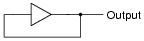What do you think this buffer will do when each input switch is separately pressed?Why does the second buffer circuit need a resistor in the feedback loop?

• #### Question 2

When studying latch circuits, you will come across many references to set and reset logic states. Give a simple definition for each of these terms in the context of latch and flip-flop circuits.

• #### Question 3

The circuit shown here is called an S-R latch: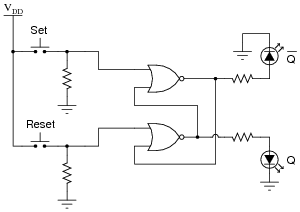Complete the truth table for this latch circuit: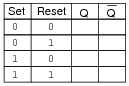• #### Question 4

Some digital circuits are considered to have active-low inputs, while others have active-high inputs. Explain what each of these terms means, and how we might identify which type of input(s) a digital circuit has.

• #### Question 5

The circuit shown here is called an S-R latch:Identify which of the two input lines is the Set, and which is the Reset, and then write a truth table describing the function of this circuit.

• #### Question 6

Latch circuits are often drawn as complete units in their own block symbols, rather than as a collection of individual gates:This simplifies schematic drawings where latches are used, much as the use of gate symbolism (as opposed to drawing individual transistors and resistors) simplifies the diagrams of more elementary digital circuits.

From the block symbols shown in this question, is there any way to determine which of the S-R latches is built with NOR gates, and which one is built with NAND gates?

• #### Question 7

The following relay logic circuit is for starting and stopping an electric motor:Draw the CMOS logic gate equivalent of this motor start-stop circuit, using these two pushbutton switches as inputs:Make sure that your schematic is complete, showing how the logic gate will drive the electric motor (through the power transistor shown).

• #### Question 8

One practical application of S-R latch circuits is switch debouncing. Explain what “bounce” refers to in mechanical switches, and also explain how this circuit eliminates it:Also, show where an oscilloscope could be connected to display any switch “bounce,” and explain how the oscilloscope would have to be configured to capture this transient event.

• #### Question 9

Complete the timing diagram, showing the state of the Q output over time as the Set and Reset switches are actuated. Assume that Q begins in the low state on power-up: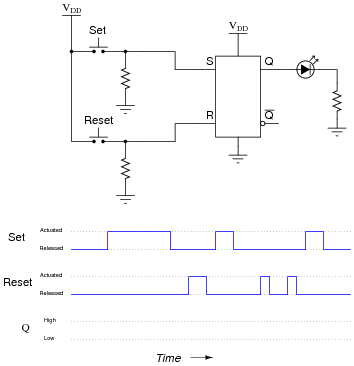• #### Question 10

A student builds this simple S-R latch for their lab experiment:When the student powers up this circuit, she notices something strange. Sometimes the latch powers up in the set state (Q high and [Q] low), and other times it powers up in the reset state (Q low and [Q] high). The power-up state of their circuit seems to be unpredictable.

What state should their circuit power up in? Did the student make an error building the latch circuit?

• #### Question 11

The circuit shown here is a gated S-R latch. Write the truth table for this latch circuit, and explain the function of the “Enable” (E) input:• #### Question 12

Here is an S-R latch circuit, built from NAND gates:Add two more NAND gates to this circuit, converting it into a gated S-R latch, with an Enable (E) input, and write the truth table for the new circuit.

• #### Question 13

Here, a gated S-R latch is being used to control the electric power to a powerful ultraviolet lamp, used for sterilization of instruments in a laboratory environment:Based on your knowledge of how gated S-R latches function, what is the purpose of the “Lockout” switch? Also, explain how the CMOS latch is able to exert control over the high-power lamp (i.e. explain the operation of the interposing devices between the latch and the lamp).

Now, suppose the lab personnel want to add a feature to the ultraviolet sterilization chamber: an electric solenoid door lock, so that personnel can open the door to the chamber only if the following conditions are met:

Lamp is off
“Lockout” switch is sending a “low” signal to the latch’s Enable input

Modify this circuit so that it energizes the door lock solenoid, allowing access to the chamber, only if the above conditions are both true.

• #### Question 14

A variation on the gated S-R latch circuit is something called the D-latch:Complete the truth table for this D latch circuit, and identify which rows in the truth table represent the set, reset, and latch states, respectively.

• #### Question 15

Complete the timing diagram, showing the state of the Q output over time as the input switches are actuated. Assume that Q begins in the low state on power-up: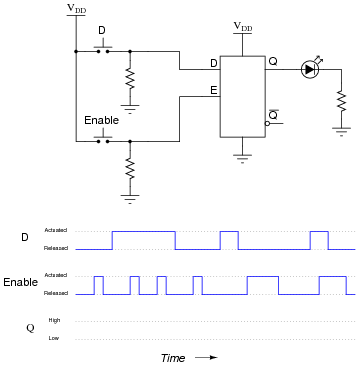• #### Question 16

Complete the timing diagram, showing the state of the Q output over time as the input switches are actuated. Assume that Q begins in the low state on power-up: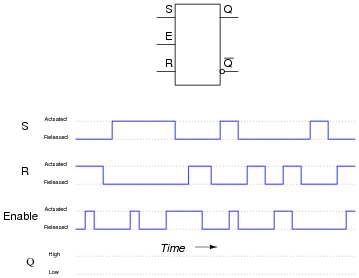• #### Question 17

An analog-to-digital converter is a circuit that inputs a variable (analog) voltage or current and outputs multiple bits of binary data corresponding to the magnitude of that measured voltage or current. In the circuit shown here, an ADC inputs a voltage signal from a potentiometer, and outputs an 8-bit binary “word,” which may then be read by a computer, transmitted digitally over a communications network, or stored on digital media: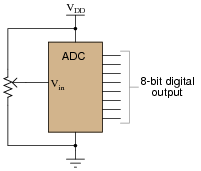As the input voltage changes, the binary number output by the ADC will change as well. Suppose, though, that we want to have sample-and-hold capability added to this data acquisition circuit, to allow us to “freeze” the output of the ADC at will. Explain how using eight D latch circuits will give us this capability: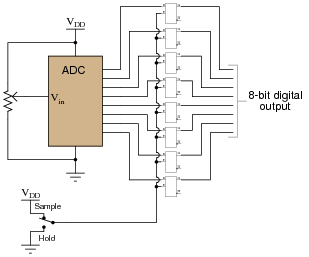• #### Question 18

Gated latch circuits often come packaged in multiple quantities, with common gate inputs, so that more than one of the latches within the integrated circuit will be enabled and disabled simultaneously. Examine this logic symbol, representative of the 74AC16373, a 16-bit D-type latch with tri-state outputs:Note how the sixteen D latches are divided into two groups of eight. Explain the functions of the four inputs at the very top of the symbol (1EN, C1, 2EN, and C2). Which of these input lines correspond to the “Enable” inputs seen on single D-type latch circuits? Also, describe what the “wedge” shapes represent on the 1EN and 2EN input lines.

Suppose you wished to have all sixteen latch circuits enabled as one, rather than as two groups of eight. Show what you would have to do to this circuit in order to achieve this goal.

• #### Question 19

In many types of digital systems, a set of square-wave signals are phase-shifted from each other by 90o. Such a phase relationship is called quadrature.

Determine the output of a D-type latch for this pair of quadrature signals, applied to the D and E inputs over time:Then, determine the output of a D-type latch when the phase relationship is reversed, (D leading E by 90o, instead of E leading D by 90o):• #### Question 20

This one-way street is equipped with an alarm to signal drivers going the wrong way. The sensors work by light beams being broken when an automobile passes between them. The distance between the sensors is less than the length of a normal car, which means as a car passes by, first one beam is broken, then both beams become broken, then only the last beam is broken, then neither beam is broken. The sensors are phototransistors sensitive only to the narrow spectrum of light emitted by the laser light sources, so that ambient sunlight will not “fool” them: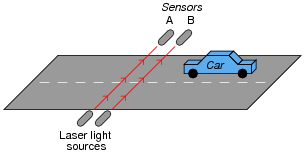Both sensors connect to inputs on a D-type latch, which is then connected to some other circuitry to sound an alarm when a car goes down the road the wrong way:The first question is this: which way is the correct way to drive down this street? From left to right, or from right to left (as shown in the illustration)?

The second question is, how will the system respond if sensor A’s laser light source fails? What will happen if sensor B’s laser light source fails?

• #### Question 21

Identify at least one component fault that would cause the “[Q]” LED to always remain off, no matter what was done with the input switches.For each of your proposed faults, explain why it will cause the described problem.

• #### Question 22

Identify at least one component fault that would cause the “Q” LED to always stay on, no matter what was done with the input switches.For each of your proposed faults, explain why it will cause the described problem.

• #### Question 23

A very common form of latch circuit is the simple “start-stop” relay circuit used for motor controls, whereby a pair of momentary-contact pushbutton switches control the operation of an electric motor. In this particular case, I show a low-voltage control circuit and a 3-phase, higher voltage motor:Explain the operation of this circuit, from the time the “Start” switch is actuated to the time the “Stop” switch is actuated. The normally-open M1 contact shown in the low-voltage control circuit is commonly called a seal-in contact. Explain what this contact does, and why it might be called a “seal-in” contact.

• #### Question 24

A student decides to build a motor start/stop control circuit based on the logic of a NOR gate S-R latch, rather than the usual simple “seal-in” contact circuit:The circuit works fine, except that sometimes the motor starts all by itself when the circuit is first powered up! Other times, the motor remains off after power-up. In other words, the power-up state of this circuit is unpredictable.

Explain why this is so, and what might be done to prevent the motor from powering up in the “run” state.

• #### Question 25

 Don’t just sit there! Build something!!

Learning to analyze relay circuits requires much study and practice. Typically, students practice by working through lots of sample problems and checking their answers against those provided by the textbook or the instructor. While this is good, there is a much better way.

You will learn much more by actually building and analyzing real circuits, letting your test equipment provide the “answers” instead of a book or another person. For successful circuit-building exercises, follow these steps:

1. Draw the schematic diagram for the relay circuit to be analyzed.
2. Carefully build this circuit on a breadboard or other convenient medium.
3. Check the accuracy of the circuit’s construction, following each wire to each connection point, and verifying these elements one-by-one on the diagram.
4. Analyze the circuit, determining all logic states for given input conditions.
5. Carefully measure those logic states, to verify the accuracy of your analysis.
6. If there are any errors, carefully check your circuit’s construction against the diagram, then carefully re-analyze the circuit and re-measure.

Always be sure that the power supply voltage levels are within specification for the relay coils you plan to use. I recommend using PC-board relays with coil voltages suitable for single-battery power (6 volt is good). Relay coils draw quite a bit more current than, say, semiconductor logic gates, so use a “lantern” size 6-volt battery for adequate operating life.

One way you can save time and reduce the possibility of error is, to begin with, a very simple circuit and incrementally add components to increase its complexity after each analysis, rather than building a whole new circuit for each practice problem. Another time-saving technique is to re-use the same components in a variety of different circuit configurations. This way, you won’t have to measure any component’s value more than once.